HELLO, GUEST## Maths Teaching Guide: Geometrical Constructions

Maths Teaching Guide: Geometrical Constructions12 Geometrical Constructions

You know

• using various instruments of the geometry box-ruler, compass, protractor, divider, set square etc.
• construction of lines and angles.
• construction of perpendicular and perpendicular bisector to a line
• construction of angle bisectors.
• Construction of special angles like 15°,30°,45°,60°,75°,90°,105°,120°,135°,150°,175°

You will learn

• construction of parallel lines using different techniques- paper folding, set square and using compass.
• to identify whether a triangle can be constructed with the given measurements.
• construction of triangles with given measurement of sides and angles.

We know parallel lines are lines that never meet. Now let us learn to construct parallel lines.

Construction of parallel lines using ruler and set squares

To construct a parallel line to a given line from a given point

Steps for construction:1.Draw a line l and take a point O outside the line.O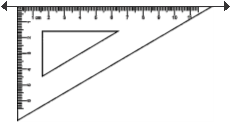2.Place any side of the set square forming the rightl

angle along the line l.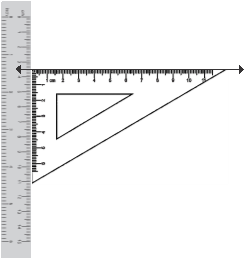3.Place the ruler along the other side of the set square forming a right angle as shown. This ruleris to be kept fixed.O

l

4.Slide the set square along the ruler upwards such that point O lies along the arm of the set square.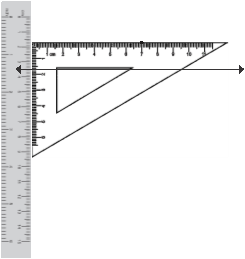O

l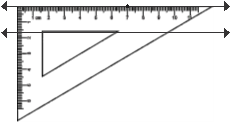5.Remove the ruler and draw a line along the setOm

square.

Name this line as ml m is the required line parallel to l

∴ l ï£¬ï£¬m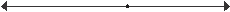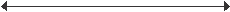Om l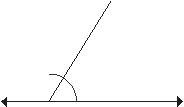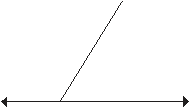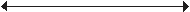Construction of parallel lines using ruler and compass

Steps for construction:

 1. Draw a line l and take a point A outside the line. A l 2. Take any point B on the line. Join A to B. A l B 3. With B as the centre and any convenient radius, draw an arc intersecting line l at P and AB at Q. A Q l BP
 4. With A as the centre and the same radius draw an arc to intersect AB at R. A Q l BP 5. With the compass measure the distance between points P and Q. 6. With R as the centre and radius equal to PQ, draw an arc intersecting the previous arc at S SA Q l BP 7. Draw a line through A and S. m is the required line parallel to l passing through the point A. l ï£¬ï£¬m SAm Q l BP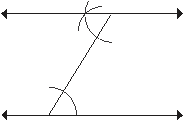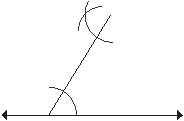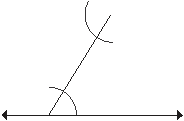Remember only one line can be drawn through A which is parallel to l.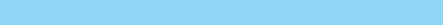Lab Activity

We have already studied parallel lines and their properties. We know that when 2 parallel lines are intersected by a transversal, the alternate angles so formed are equal. The above construction has been done using the same property.

When 2 parallel lines are intersected by a transversal, then the corresponding angles so formed are also equal. Using this property, construct a pair of parallel lines.

To construct a parallel line to a given line at a given distance

To draw a parallel line at a fixed distance from a given line follow the steps given below

1. Draw line l.
2. Construct a perpendicular on the given line.
3. Take a point at the given distance on the perpendicular.
4. Construct a parallel line at that point as in the previous construction.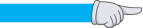Example 1Draw a line l. Draw another line m parallel to l at a distance of 4 cm from it.

Solution

To construct a line parallel to a given line at a fixed distance from it we

1. Take a point C on the line l.
2. Draw a perpendicular at the point C.
3. On the perpendicular mark a point at a distance of 4 cm from C (say G).
4. At G draw a GH perpendicular to CG.

Since GH ⊥ CG and CG ⊥ l

∴ l ï£¬ï£¬ GH

GHmF

DE

l

ACB

(since the sum of the interior angles on the same side of the transversal CG is 180°) Thus, m ï£¬ï£¬ l at a distance of 4 cm from l.Exercise 12.1

1.Draw a line AB = 6 cm. Mark a point P anywhere outside the line AB. Draw a line CD parallel to line

AB passing through the point P

a. by drawing alternate angles

b. by drawing corresponding angles.

2. Draw a line AB. Draw a line CD perpendicular to line AB. Now on CD mark a point P at a distance of 4.5 cm from C. At the point P draw a line parallel to given line AB.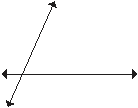3. Refer to the figure given alongside. Construct a line parallel to AB passingD through the point P. Draw another line parallel to CD also through thepoint P. Name the geometrical plane figure so formedP

AB

C

5. Draw a line XY= 8 cm. On the line XY mark a point A, 3 cm from X. At the point A draw a perpendicular AB to the line XY. Mark a point M on AB at a distance of 4 cm from A. draw a line CD parallel to XY passing through M.

6. Draw a line parallel to a given line at a distance of 5.5 cm from it.

Construction of triangles

A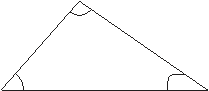A triangle is a three sided closed figure. It has 6 elements -3 sides and

3 angles.

For triangle ABC given alongside, sides are AB, BC, and CA and the angles are ∠ABC, ∠BCA and ∠CBA

However to construct a triangle uniquely, we do not need the measure

of all six parts. A triangle can be drawn with a definite given size if any BC

of the 3 conditions given below are fulfilled.

ÂŠ The three sides of the triangle are given – SSS criterion

• Two sides and the included angle are given – SAS criterion.
• One side and any two angles are given – AAS criterion or ASA criterion.

Use a compass to draw angles of special measures – 15°, 30°, 45°, 60°, 75°, 90°, 105°, 120°, 135° etc). For others you can use a protractor to construct triangles with given angles.

Remember: A triangle cannot be constructed if• 3 angles are given since the length of sides can vary. The triangles will be of the same shape; however the length of the sides will be different.
• Two sides and the non – included angles are given.

Before we construct triangles we should make a rough sketch showing all the given measures.

Construction of triangles when 3 sides are given.

A triangle can be drawn only when the sum of any two sides is greater than the third side.

When three sides of a triangle are given, check whether the sum of any two sides is greater than the third side.

If yes, only then the construction is possible.Example 1Which of the following can be the sides of a triangle?

a.12,24, 11b.10, 5, 7

Solution

a.Add the sides by taking two at a time

12 + 24 > 11

24 + 12 > 15

However 11+12 < 24, hence these measures cannot be the sides of a triangle b.Add the sides by taking two at a time

10 + 5 >7

5 + 7 >10

10 + 7 >5

Since the sum of any two sides is greater than the third side hence these measures can be the measures of a triangle.Example 2Construct a triangle ABC such that AB = 6 cm, BC = 5 cm and CA = 9 cm.

Solution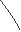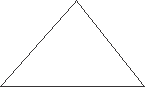In triangle ABC, 9 + 6 > 5, 6 + 5 > 9, 9 + 5 > 6 ∴ triangle ABC can be constructed.

Steps of Construction

 Draw a rough sketch of the triangle ABC. C 9 cm5 cm A6 cmB Draw a line segment AB = 6 cm A6 cmB With A as the centre and radius = AC=9 cm draw an arc A6 cmB
 With B as centre and radius = BC= 5 cm draw another arc to intersect the previous arc at C A6 cmB Join A to C and B to C. Triangle ABC is the required triangle. C 9 cm 5 cm A6 cmB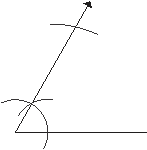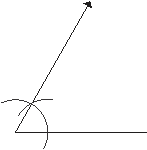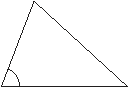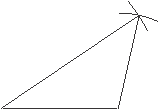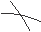Example 3Construct a triangle PQR with PQ = 7 cm, QR = 6 cm and ∠PQR = 60°.

Solution:

Steps of Construction

 Draw a rough sketch of the triangle PQR R 6 cm 60â-¦ P7 cmQ Draw a line segment PQ of measure 7 cm. P7 cmQ Using a protractor or a compass construct an angle of 60° at the point P. X 60° P7 cmQ With P as the centre and the radius = PR = 6 cm draw an arc to intersect XP at a point R X R 6 cm 60° P7 cmQ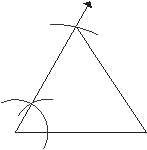Join RQ.X Triangle PQR is the required triangle. R

6 cm

60°

P7 cmQ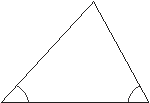To construct a triangle when two angles and the included sides are given- ASA construction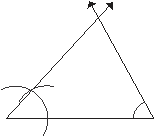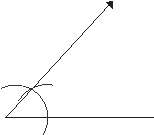Example 4Construct a triangle ABC with ∠B = 60°, ∠C = 70° and BC = 8 cm.

 Draw a rough sketch of the triangle ABC A 6 cm 60°70° B8 cmC Draw a line segment BC of length = 8 cm B8 cmC At B draw ∠PBC = 60° using a compass P 60° B8 cmC At C draw ∠QCB = 70° using a protractor the point off intersection of PB and QC is the vertex A. Triangle ABC is the required triangle. QP A 6 cm 60°70° B8 cmC

To construct a triangle when two angles and the side not included between the angles is given- AAS

construction

To construct a triangle when the side is not the included side in the given angles, we will first the third angle using the angle sum property and then consider the given side and the two angles that include that side to construct the triangle using ASA construction criterion.Example 5Construct a triangle PQR with ∠P = 110°, ∠Q= 30° and QR = 6.5 cm.

Solution:

The given side QR is not the included side between the given angles ∠P and ∠ Q.

∴ let us find the third angle ∠R, using the angle sum property

We know sum of angles of a triangle = 180°.

∠P + ∠ Q + ∠R = 180°

⇒ 110° + 30° +∠R = 180°

⇒ ∠R = 180° – 140° = 40°

Now we can use the ASA construction criterion to construct triangle PQR with ∠Q =30°, ∠R = 40° and

QR = 6.5 cm.

The steps of construction will be the same as in the previous construction

Rough sketch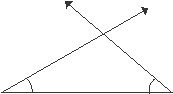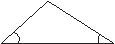PAB P

30°

40°

Q6.5 cmR

30°40°

Q6.5 cmR

To construct a right triangle when the hypotenuse and one side are given.RHS construction

This construction is only for right angled triangles when the hypotenuse and one side are given. One angle is

90° as it is a right triangle.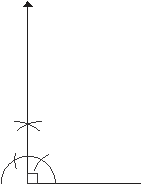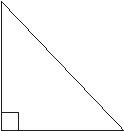Example 6Construct a right triangle XYZ right angled at X with hypotenuse YZ = 5 cm and XY =

3 cm

Solution:

Since it is a right triangle right angled at X

∴ ∠X = 90°, YZ = 5 cm and XY = 3

Steps of construction

 Draw a rough sketch of the triangle XYZ Z 5 cm X3 cmY Draw a line segment XY = 3 cm. X3 cmY At X draw ∠AXY = 90° using a compass A 90° X3 cmY
 With Y as the centre and radius 5 cm , draw an arc to intersect AX at Z. A Z 90° X3 cmY Join YZ Triangle XYZ is the required triangle. A Z 5 cm 90° X3 cmY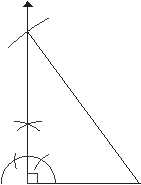Remember in a right triangle, the hypotenuse is the longest side.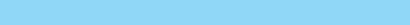Exercise 12.3

1. Given below are some measurements of sides, which of the following can be the sides of a triangle. a. 6,8,12 b. 5,9,6 c. 11,6,6 d. 80,15,60 e. 8,6,10 f. 6,6,6

2. Which of the following measures will form a triangle? Why or why not?

 a.∠A = 45°, ∠B = 80°, ∠C = 65° b.∠X = 30°, XY = 5.6 cm, XZ = 3.8 cm c.AB = 7 cm, BC = 10 cm, CA = 6 cm d.∠B = 60°, ∠A = 80°, AC = 5 cm

2. Construct a triangle ABC with each side measuring 6 cm. Measure the three angles of the triangle so formed.

3. Construct a right triangle PQR right angled at P with PQ = 4 cm and PR = 6 cm.

4. Construct a triangle XYZ with ∠X = 60°, ∠Y = 45° and XY = 7 cm.

5. Construct a triangle PQR with PQ = 6 cm, PR = 8 cm and ∠Q = 75°.

6. Construct a triangle ABC with AB = 5 cm, BC = 6 cm, ∠B = 105°

7. Construct a triangle LMN with LM = LN = 5.8 cm, MN = 4. 6. What special name is given to such a triangle?

8. Construct a right triangle ABC with AB = 5.5 cm, BC =8.5 cm and ∠A = 90°

9. Construct a triangle PQR with ∠P = 45°, ∠Q = 75° and PQ = 5.5 cmConstruct a triangle PQR with measures of sides PQ = 4.6 cm, QR = 5.6 cm and PR = 6.5 cm.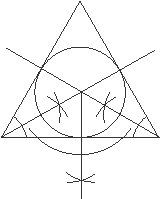1.Draw the angle bisectors of ∠P and ∠Q. let these intersect each otherR

at the point O.

2. From the point O draw a perpendicular to any side of the triangle. Name the point where it meets the side as M.

3. With O as the centre and radius OM draw a circle.O Does the circle touch all the sides of the triangle?

Such a circle is called an inscribed circle and the centre is known as the incentre.PMQ

Can you draw another circle larger than this which can fit into the triangle? No the inscribed circle is the largest circle that will fit inside the triangle.Math Lab Activity

Objective: to make students familiar with constructions

Materials required: compass, ruler, paper, pencil and colours.

Method: Each student will work individually to create a drawing of his/her initials using the parallel, perpendicular, and segment bisector constructions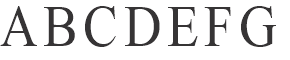1.Make a sketch of your initials and identify where each construction will be used.

It is necessary to use at least one

Š perpendicular line through a point on a line,

Š perpendicular line through a point not on a line,

Š parallel line through a point not on the line, other constructions what you have learned can

H I J K L M N

Š

also be used.

2.Construct using a compass and a ruler.

3.Colour the alphabets and make them as creative as you can.

Hint: constructions will be easy if you use the straight lined alphabets asRecollections

OPQRSTU

V W X Y Z

• A parallel line can be drawn to a given line from a given point
• A parallel line to a given line can be drawn at a given distance from it.
• A triangle has 6 elements in all- 3 sides and 3 angles.
• A triangle is possible only if the sum of any 2 sides is greater than the third side.
• Construction of triangles is possible given the following criterions
• when 3 sides are given. SSS
• when two sides and an included angle are given.SAS
• when two angles and the included sides are given.ASA construction
• when two angles and the side not included between the angles is given. AAS construction
• a right triangle when the hypotenuse and one side are given. RHS construction

Formative assessment

1.Fill in the blanks

a.The sum of angles of a triangle is . b.A triangle has elements.

c.If 2 angles and the side are given, a triangle can be constructed.

d.In a triangle PQR, ∠ P = 45°, PQ = 7.5 cm and PR = 6.3 cm, then triangle PQR can be constructed using criterion.

e.To construct a triangle with given sides, the sum of 2 sides should be than the third side.

2.Which of the following can be the sides of a triangle?

a.4 cm, 6 cm, 5 cm.b.2 cm, 5 cm, 4 cmc.8 cm, 6 cm, 12 cm d.5 cm, 6 cm, 12 cm

3.Construct a triangle ABC with the following measurements:

a.AB = 5 cm, BC = 7 cm, AC = 13 cm.b.∠A = 45°, ∠B= 65°, AB = 7 cm.

4.Draw a line parallel to a given line at a distance of 7.5 cm from it.

5.How many lines parallel to a given line can be drawn through a point outside the line? Why?

Review Exercise

1.Draw a line segment AB = 6.4 cm. On AB take any point P. At P draw perpendicular PQ to AB. On PQ mark a point at 5 cm from P. Draw a line parallel to given line AB.

2.Draw a right triangle PQR right angled at Q with PQ = 7 cm , QR = 6 cm. through P draw a line parallel to QR and through R draw a line parallel to PQ intersecting each other at S. measure PS and RS. What is the name of the figure so obtained?

3.Construct an isosceles triangle ABC with AB = AC= 7.5 cm and ∠A = 75°.

4.Construct an equilateral triangle LMN with each side measuring 6 cm.

5.Construct a right triangle XYZ with XY = 6.5 cm, YZ =8.5 cm and ∠X = 90°.

6.Construct an obtuse triangle ABC with ∠B = 135 °, AB = 7 cm, BC = 8 cm.

7.Construct a triangle PQR with ∠P = 55°, ∠Q = 65° and PQ = 6.3 cm

8.Construct a triangle ABC with ∠A = ∠B =75°, and AB = 7.4 cm. What is the special name given to such a triangle?

9.Construct a triangle XYZ with XY = 5.4 cm and ∠X=60°, ∠Z = 60°. Measure the length of YZ and XZ. What is the special name given to such a triangle?

10.Construct a triangle ABC with the ∠B = 105°, AB= 6.3 cm and BC = 5.6 cm.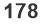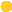## Most Used Categories

Testimonials
I order from this writer for quite a while, so we are having the chemistry going on between us. Great job as always!
Laura C., March 2018
Wow, ordering from EssayHub was one of the most pleasant experiences I have ever had. Not only was my work sent to me hours before the deadline, but the content was absolutely fantastic! Would order from them again!
Daniel L., March 2018
Professional Custom
Professional Custom Essay Writing Services
In need of qualified essay help online or professional assistance with your research paper?
Browsing the web for a reliable custom writing service to give you a hand with college assignment?
Out of time and require quick and moreover effective support with your term paper or dissertation?